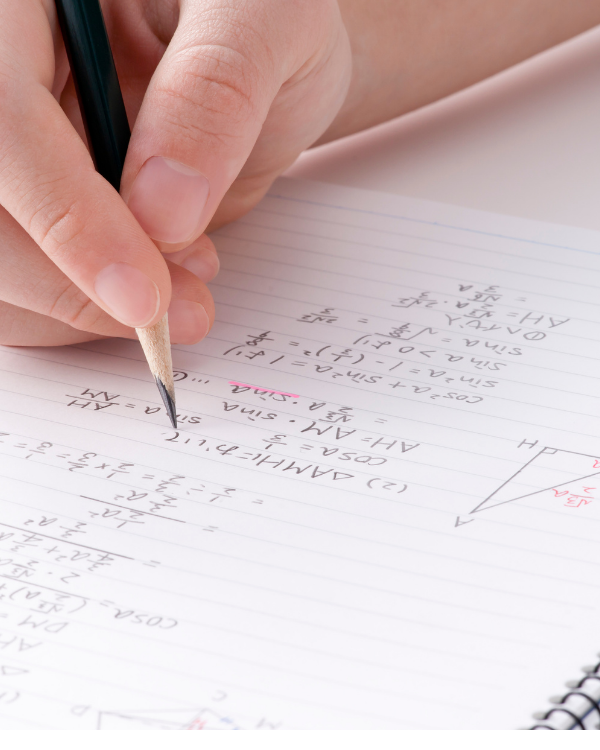# A LEVEL MATHEMATICSOverviewExamsFurther Progression

## Course Overview

The International A Level Mathematics course is spread out over two years with the possibility for an Advanced Subsidiary (AS) Level or Advanced (A) Level qualification.

For the AS Level qualification you have to take three units - two compulsory units of Pure Mathematics, and one of either Mechanics, Statistics or Decision Mathematics.

For the A Level qualification you have to take six units - four of Pure Mathematics, and two as a combination of Mechanics, Statistics and Decision Mathematics.

### Students will learn to:

• Develop their understanding of mathematics and mathematical processes in a way that promotes confidence and fosters enjoyment
• Develop abilities to reason logically and recognise incorrect reasoning, to generalise and to construct mathematical proofs
• Extend their range of mathematical skills and techniques and use them in more difficult, unstructured problems
• Develop an understanding of coherence and progression in mathematics and of how different areas of mathematics can be connected
• Recognise how a situation may be represented mathematically and understand the relationship between ‘real-world’ problems and standard and other mathematical models and how these can be refined and improved
• Use mathematics as an effective means of communication
• Read and comprehend mathematical arguments and articles concerning applications of mathematics
• Acquire the skills needed to use technology such as calculators and computers effectively, recognise when such use may be inappropriate and be aware of limitations
• Develop an awareness of the relevance of mathematics to other fields of study, to the world of work and to society in general • take increasing responsibility for their own learning and the evaluation of their own mathematical development.## Exam Overview

The Pearson Edexcel International A Level qualification in Mathematics offers external assessment for each of the 9 possible units of study.

##### Duration

AS Level: Three units of 1 hour and 30 minutes each A Level: Six units of 1 hour and 30 minutes each

##### Format

External assessment offered three times a year for each of the nine units.

##### Components

AS Level | 3 units of 75 marks each A Level | 6 units of 75 marks each

Created By CGA
Created By CGA